# Test Prep Plan - Take a practice test

Take this practice test to check your existing knowledge of the course material. We'll review your answers and create a Test Prep Plan for you based on your results.
How Test Prep Plans work
1
2Based on your results, we'll create a customized Test Prep Plan just for you!
3Study smarter
Study more effectively: skip concepts you already know and focus on what you still need to learn.

# Definite Integrals Chapter Exam

Exam Instructions:

Choose your answers to the questions and click 'Next' to see the next set of questions. You can skip questions if you would like and come back to them later with the yellow "Go To First Skipped Question" button. When you have completed the practice exam, a green submit button will appear. Click it to see your results. Good luck!

### Page 1

#### Question 1 1. Which of the following represents the area of the FIRST rectangle being used to estimate the area under the curve f(x) between x = 0 and x = 2 when divided into 4 slices?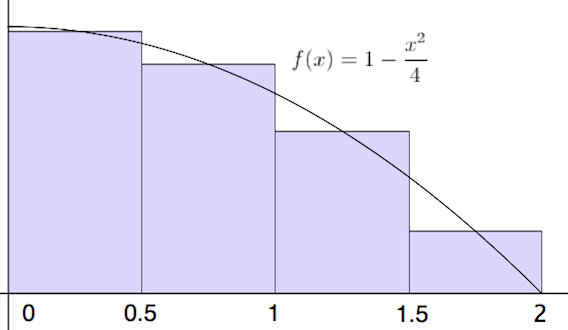#### Question 2 2. How many terms are in the Riemann sum represented below?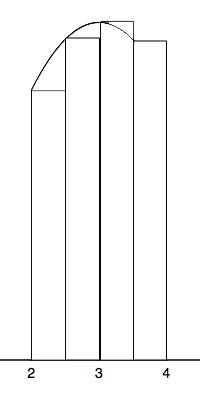#### Question 3 3. You are going to take a Riemann Sum of the area below. To do this, you divide the region into four slices of equal width. How wide is each slice?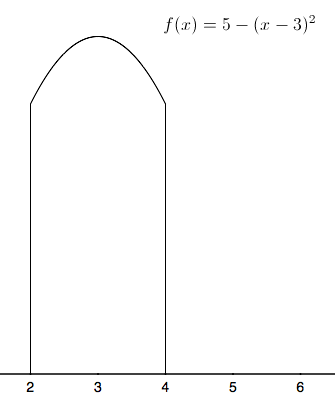#### Question 4 4. Using the single term Riemann sum as shown, what is the area beneath the curve f(x) between 0 and two pi?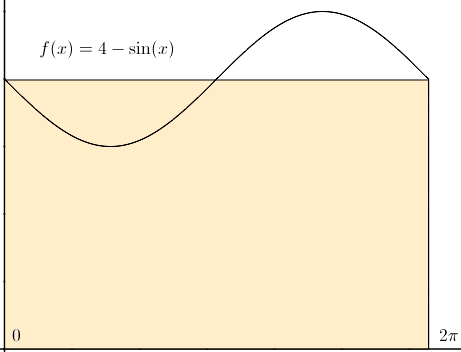#### Question 5 5. Estimate the area beneath the curve f(x) using the two term Riemann sum as shown below.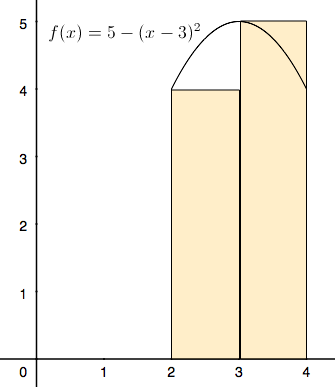### Page 3

#### Question 11 11. Which of the following integrals represents the area under the graph below?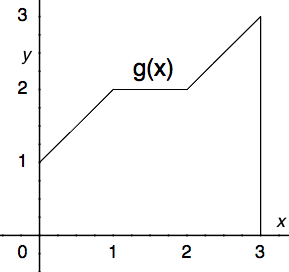#### Question 13 13. Which of the following graphs shows the correct region of integration for the integral below?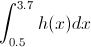#### Question 14 14. Given f(x) on the graph, what does z equal?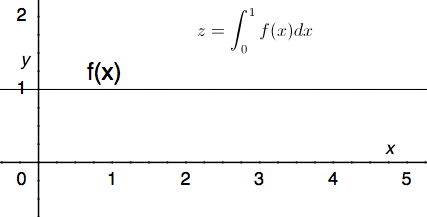#### Question 15 15. Given f(x) on the graph below, which of the following integrals will equal 0?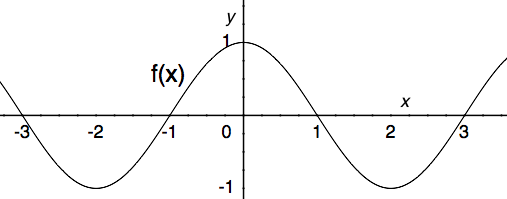### Page 4

#### Question 16 16. Given a continuous function f(x) that satisfies the equation below, which of the following is true, according to the average value theorem?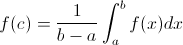#### Question 17 17. The average value for this continuous function over the shaded region is 2. According to the average value theorem, how many times does this function pass through the value 2?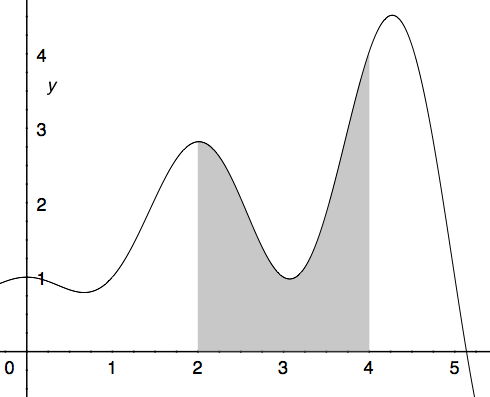#### Question 20 20. Use the average value theorem to determine the average value of f(x) between 0 and 2.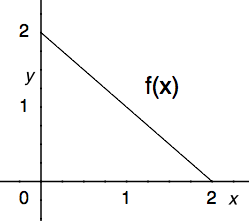### Page 5

#### Definite Integrals Chapter Exam Instructions

Choose your answers to the questions and click 'Next' to see the next set of questions. You can skip questions if you would like and come back to them later with the yellow "Go To First Skipped Question" button. When you have completed the practice exam, a green submit button will appear. Click it to see your results. Good luck!

Support